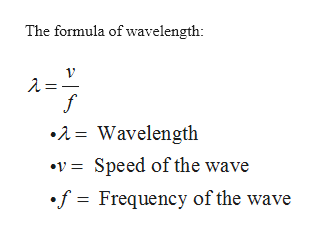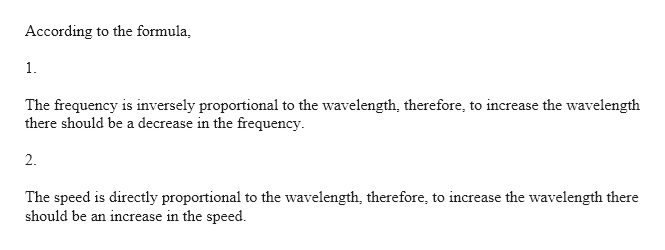# Q/ The wavelength of a wave in a medium increases as a result of which of the following changes in parameters? A. an increase in frequency B. a decrease in frequency C.an increase in speed D. a decrease in speed

Question
4 views

Q/ The wavelength of a wave in a medium increases as a result of which of the following changes in parameters?

A. an increase in frequency

B. a decrease in frequency

C.an increase in speed

D. a decrease in speed

check_circle

Step 1help_outlineImage TranscriptioncloseThe formula of wavelength: •1= Wavelength •v = Speed of the wave •f = Frequency of the wave fullscreen
Step 2help_outlineImage TranscriptioncloseAccording to the formula, 1. The frequency is inversely proportional to the wavelength, therefore, to increase the wavelength there should be a decrease in the frequency. 2. The speed is directly proportional to the wavelength, therefore, to increase the wavelength there should be an increase in the speed. fullscreen

### Want to see the full answer?

See Solution

#### Want to see this answer and more?

Solutions are written by subject experts who are available 24/7. Questions are typically answered within 1 hour.*

See Solution
*Response times may vary by subject and question.
Tagged in

### Other